PEMDAS
Dividing Complex Fractions
Number/Written Properties /Descriptions
Exponent Same Base
Miscellaneous
100What is 22

100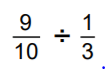What is 27/10

100
Three more than x

What is x + 3

100

Simplify a4 * a3

What is a7

100

What is the name of Ariel's fish friend?

Who is Flounder?

200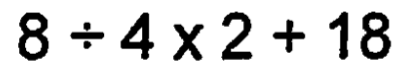What is 22

200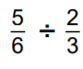What is 5/4

200

The difference between 5 and y2

What is 5 - y2

200

Simplify a9/a2

What is a7

200

Happy, Sleepy, Sneezy, Grumpy, Dopey, Doc, and ________?

Who is Bashful?

300What is 63

300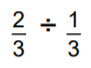What is 2 or 2/1

300
Commutative property (complete)

x + 5 =

What is 5 + x

300

Simplify 2b* 3b5

What is 6b9

300

What is Goku's real name?

What is Kakarat?

400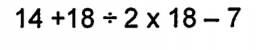What is 169

400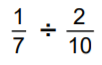What is 5/7

400

The product 13 and 2x

(simplify if possible)

What is 26x

400

Simplify (1234ab)0

What is 1

400

What is Platform 9 and 3/4?

500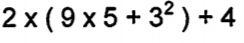What is 112

500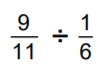What is 54/11

500

The reciprocal of -2 is...

What is -1/2

500

Simplify m4/m10

What is 1/m6

500

Nezuko is half what?

What is either half-human or half-demon?

Click to zoom